SPSS TUTORIALS BASICS ANOVA REGRESSION FACTOR CORRELATION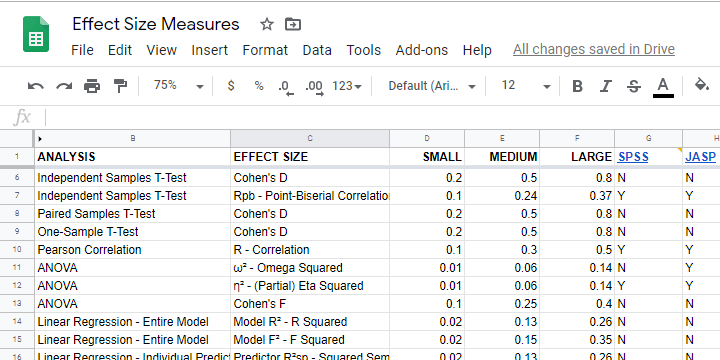### Effect Size – A Quick Guide

Effect size measures for all major analyses with rules for small, medium and large effects and more...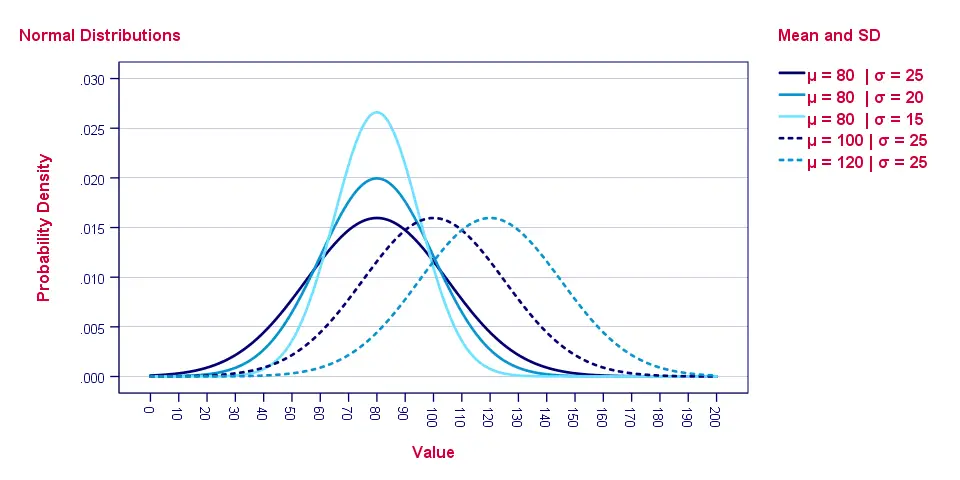### Normal Distribution – Quick Intro

The ultimate introduction to normal distributions!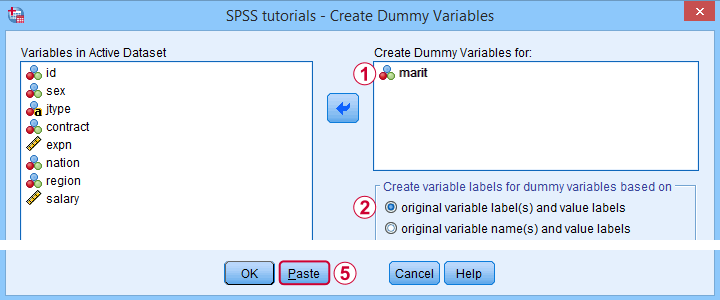### SPSS – Create Dummy Variables Tool

Create dummy variables for regression straight from the menu!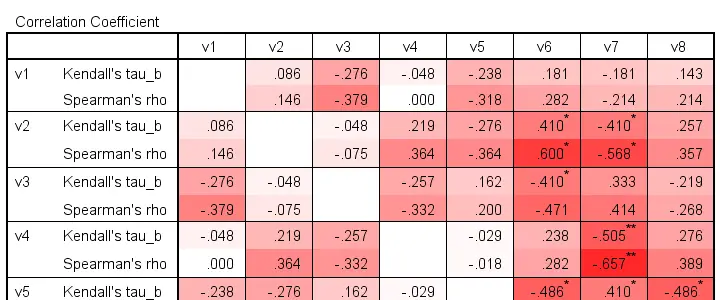### Kendall’s Tau – Simple Introduction

Quickly read up on this correlation for ordinal and quantitative variables!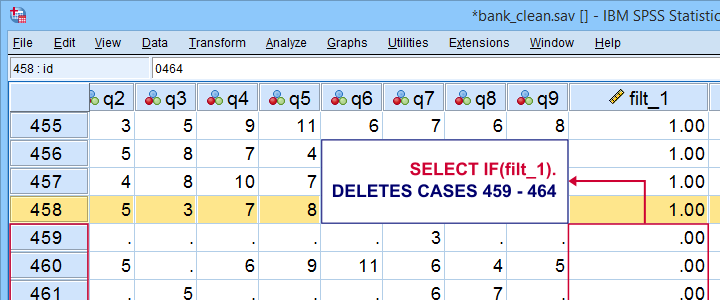### SPSS SELECT IF – Tutorial & Examples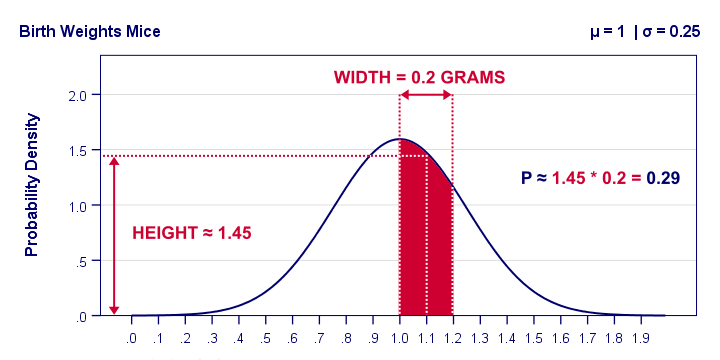### Probability Density Functions

A Quick and Simple Introduction!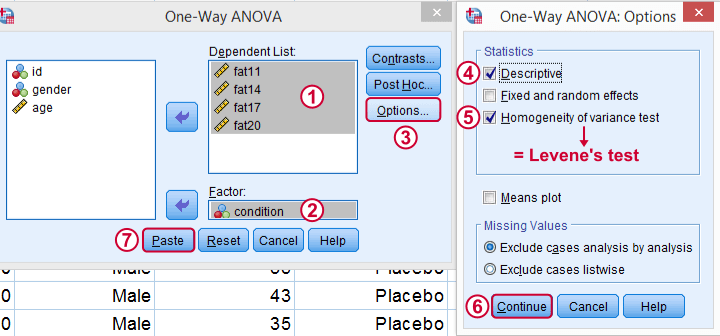### How to Run Levene’s Test in SPSS?

Levene's test evaluates the homogeneity of variances assumption required by ANOVA. This tutorial quickly walks you through.